# Conditional Statements and Conditional Statements Biconditional Statements Warm

• Slides: 60Conditional Statements and Conditional Statements Biconditional Statements Warm Up Lesson Presentation Lesson Quiz Holt Mc. Dougal GeometryConditional Statements Warm Up Determine if each statement is true or false. 1. The measure of an obtuse angle is less than 90°. 2. All F perfect-square numbers are positive. T 3. Every prime number is odd. 4. Any three points are coplanar. F T Holt Mc. Dougal GeometryConditional Statements Objectives Identify, write, and analyze the truth value of conditional statements. Write the inverse, converse, and contrapositive of a conditional statement. Holt Mc. Dougal GeometryConditional Statements Vocabulary conditional statement hypothesis conclusion truth value negation converse inverse contrapostive logically equivalent statements Holt Mc. Dougal Geometry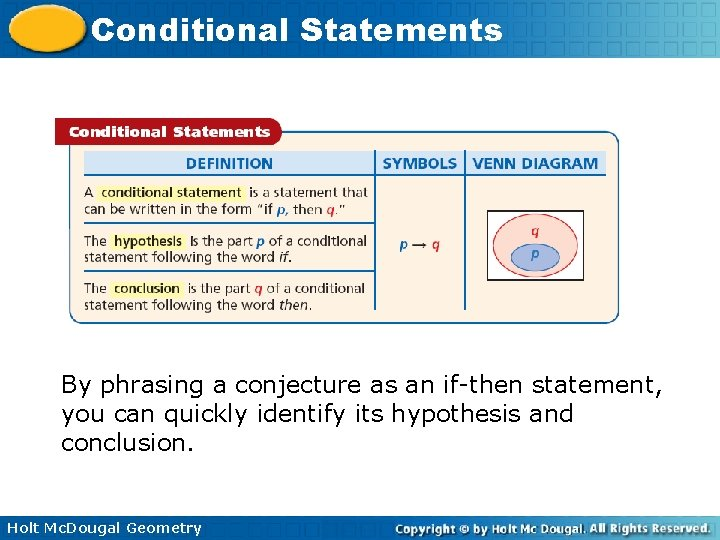Conditional Statements By phrasing a conjecture as an if-then statement, you can quickly identify its hypothesis and conclusion. Holt Mc. Dougal Geometry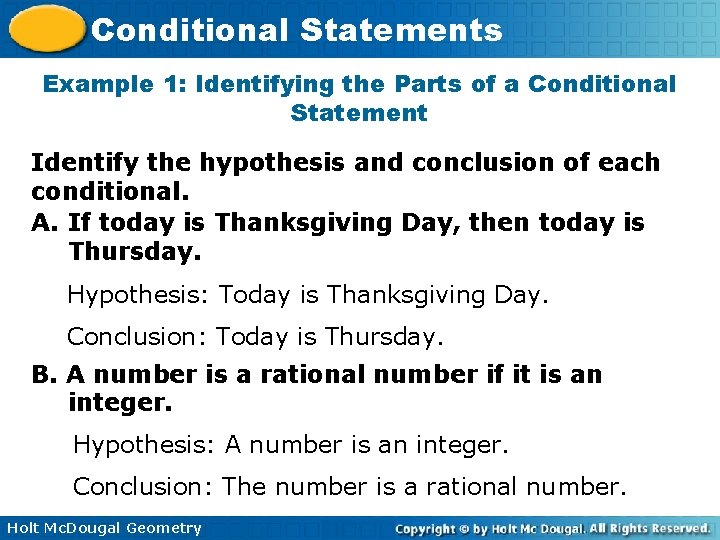Conditional Statements Example 1: Identifying the Parts of a Conditional Statement Identify the hypothesis and conclusion of each conditional. A. If today is Thanksgiving Day, then today is Thursday. Hypothesis: Today is Thanksgiving Day. Conclusion: Today is Thursday. B. A number is a rational number if it is an integer. Hypothesis: A number is an integer. Conclusion: The number is a rational number. Holt Mc. Dougal Geometry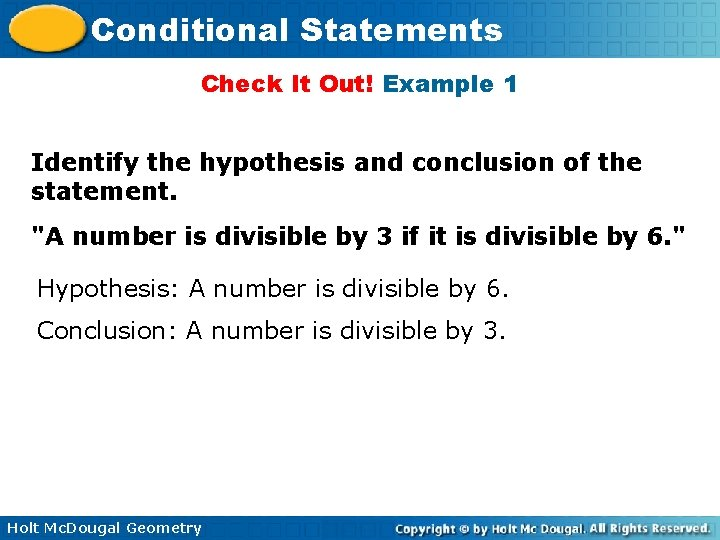Conditional Statements Check It Out! Example 1 Identify the hypothesis and conclusion of the statement. "A number is divisible by 3 if it is divisible by 6. " Hypothesis: A number is divisible by 6. Conclusion: A number is divisible by 3. Holt Mc. Dougal Geometry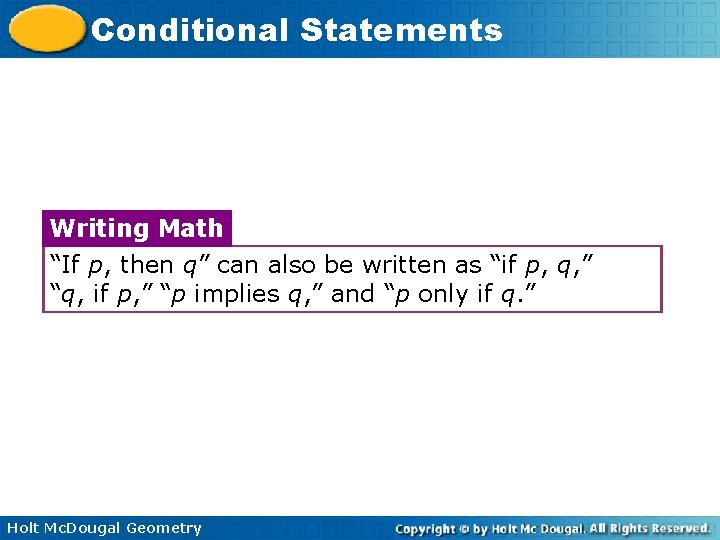Conditional Statements Writing Math “If p, then q” can also be written as “if p, q, ” “q, if p, ” “p implies q, ” and “p only if q. ” Holt Mc. Dougal Geometry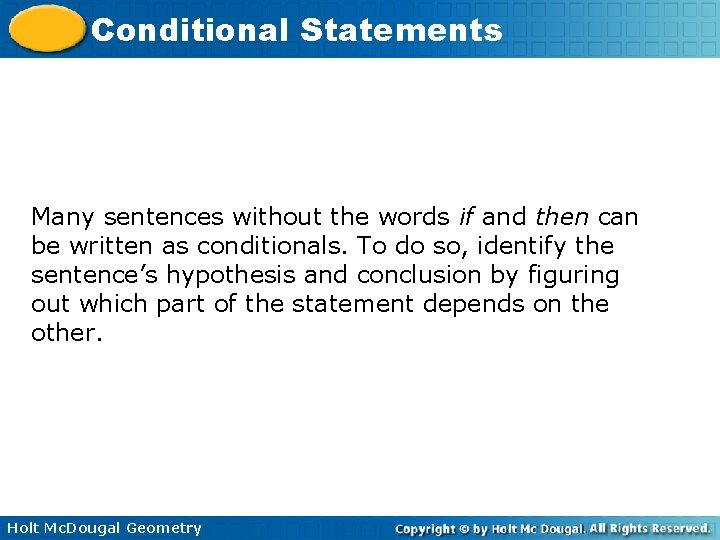Conditional Statements Many sentences without the words if and then can be written as conditionals. To do so, identify the sentence’s hypothesis and conclusion by figuring out which part of the statement depends on the other. Holt Mc. Dougal Geometry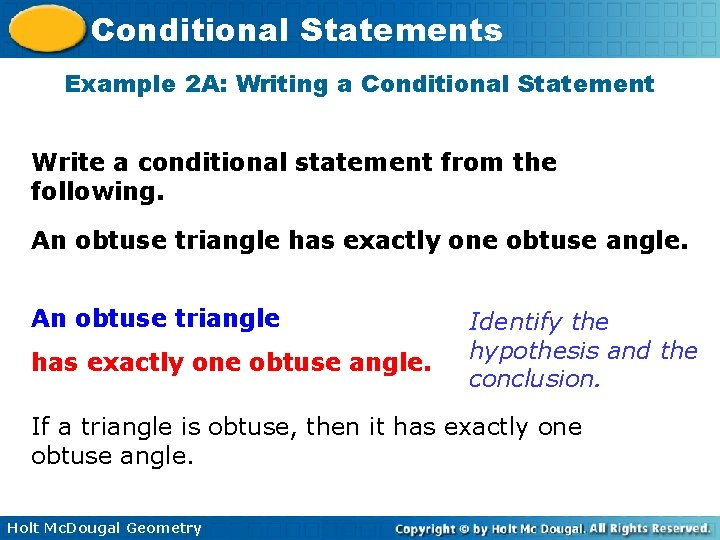Conditional Statements Example 2 A: Writing a Conditional Statement Write a conditional statement from the following. An obtuse triangle has exactly one obtuse angle. Identify the hypothesis and the conclusion. If a triangle is obtuse, then it has exactly one obtuse angle. Holt Mc. Dougal Geometry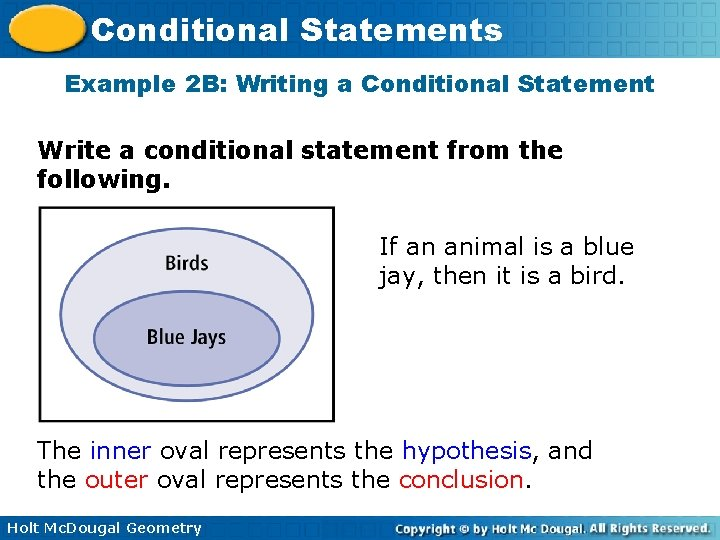Conditional Statements Example 2 B: Writing a Conditional Statement Write a conditional statement from the following. If an animal is a blue jay, then it is a bird. The inner oval represents the hypothesis, and the outer oval represents the conclusion. Holt Mc. Dougal GeometryConditional Statements Check It Out! Example 2 Write a conditional statement from the sentence “Two angles that are complementary are acute. ” Two angles that are complementary are acute. Identify the hypothesis and the conclusion. If two angles are complementary, then they are acute. Holt Mc. Dougal GeometryConditional Statements A conditional statement has a truth value of either true (T) or false (F). It is false only when the hypothesis is true and the conclusion is false. To show that a conditional statement is false, you need to find only one counterexample where the hypothesis is true and the conclusion is false. Holt Mc. Dougal GeometryConditional Statements Example 3 A: Analyzing the Truth Value of a Conditional Statement Determine if the conditional is true. If false, give a counterexample. If this month is August, then next month is September. When the hypothesis is true, the conclusion is also true because September follows August. So the conditional is true. Holt Mc. Dougal Geometry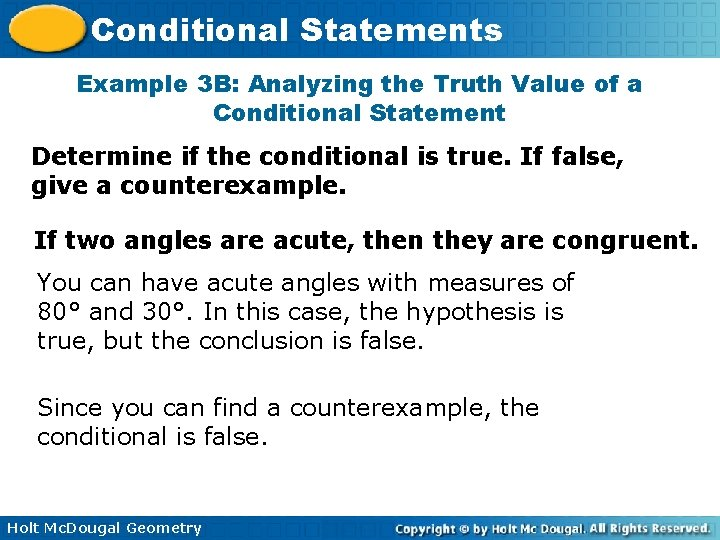Conditional Statements Example 3 B: Analyzing the Truth Value of a Conditional Statement Determine if the conditional is true. If false, give a counterexample. If two angles are acute, then they are congruent. You can have acute angles with measures of 80° and 30°. In this case, the hypothesis is true, but the conclusion is false. Since you can find a counterexample, the conditional is false. Holt Mc. Dougal GeometryConditional Statements Example 3 C: Analyzing the Truth Value of a Conditional Statement Determine if the conditional is true. If false, give a counterexample. If an even number greater than 2 is prime, then 5 + 4 = 8. An even number greater than 2 will never be prime, so the hypothesis is false. 5 + 4 is not equal to 8, so the conclusion is false. However, the conditional is true because the hypothesis is false. Holt Mc. Dougal Geometry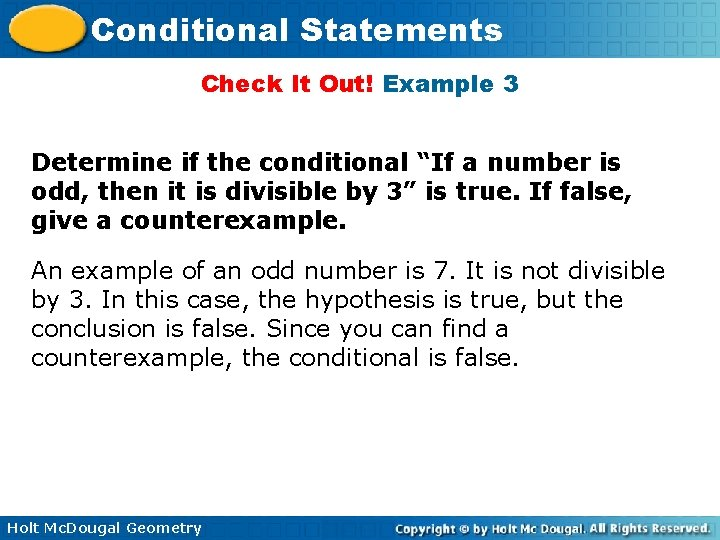Conditional Statements Check It Out! Example 3 Determine if the conditional “If a number is odd, then it is divisible by 3” is true. If false, give a counterexample. An example of an odd number is 7. It is not divisible by 3. In this case, the hypothesis is true, but the conclusion is false. Since you can find a counterexample, the conditional is false. Holt Mc. Dougal Geometry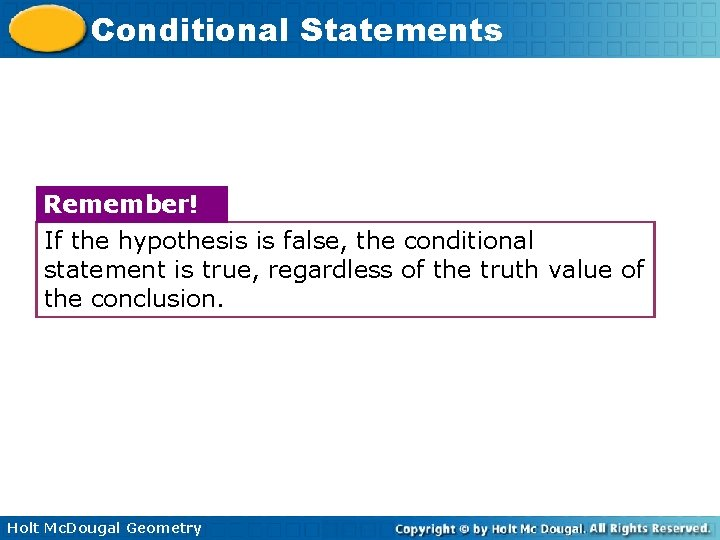Conditional Statements Remember! If the hypothesis is false, the conditional statement is true, regardless of the truth value of the conclusion. Holt Mc. Dougal Geometry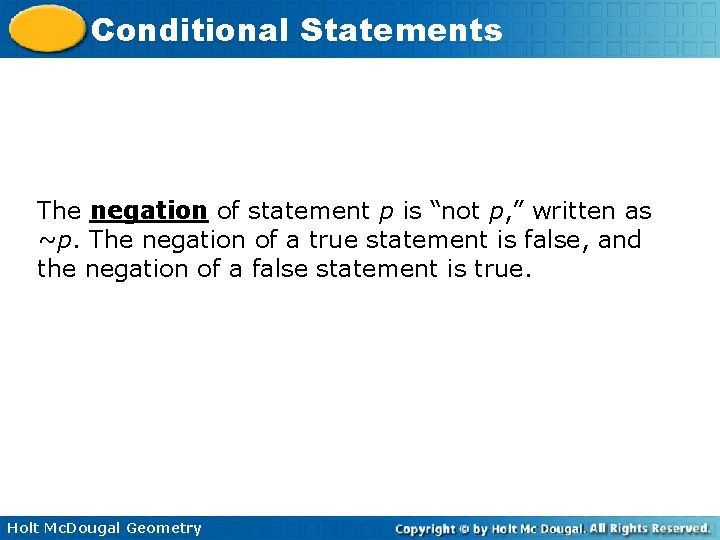Conditional Statements The negation of statement p is “not p, ” written as ~p. The negation of a true statement is false, and the negation of a false statement is true. Holt Mc. Dougal GeometryConditional Statements Related Conditionals Definition A conditional is a statement that can be written in the form "If p, then q. " Holt Mc. Dougal Geometry Symbols p qConditional Statements Related Conditionals Definition The converse is the statement formed by exchanging the hypothesis and conclusion. Holt Mc. Dougal Geometry Symbols q p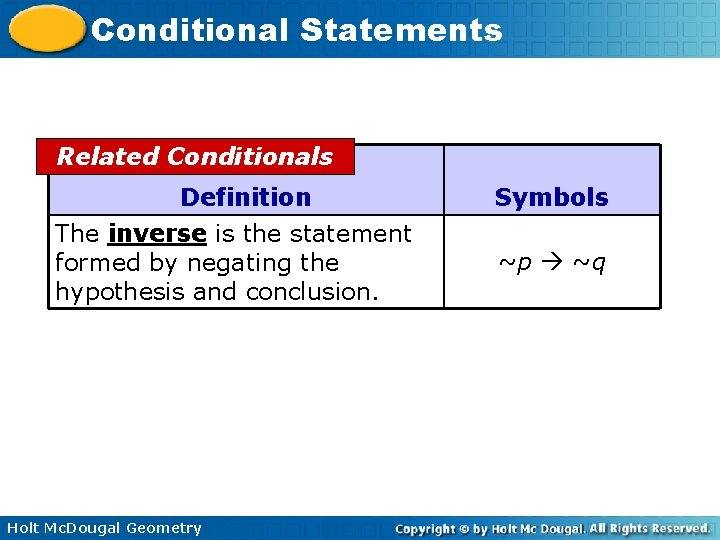Conditional Statements Related Conditionals Definition The inverse is the statement formed by negating the hypothesis and conclusion. Holt Mc. Dougal Geometry Symbols ~p ~qConditional Statements Related Conditionals Definition The contrapositive is the statement formed by both exchanging and negating the hypothesis and conclusion. Holt Mc. Dougal Geometry Symbols ~q ~p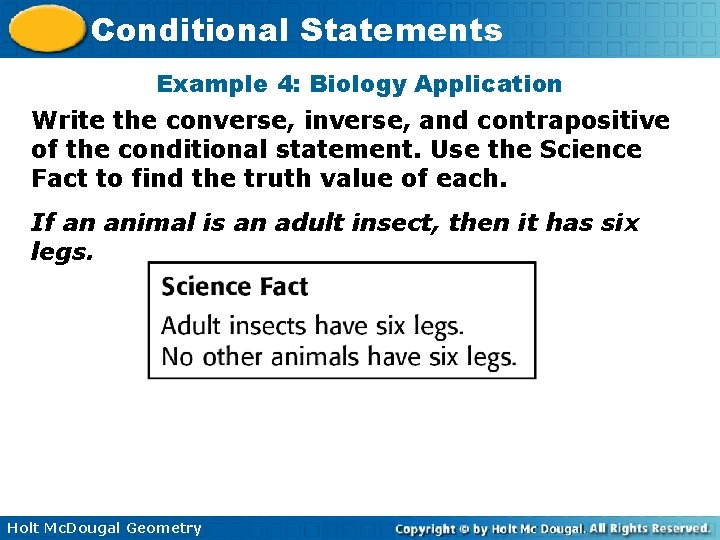Conditional Statements Example 4: Biology Application Write the converse, inverse, and contrapositive of the conditional statement. Use the Science Fact to find the truth value of each. If an animal is an adult insect, then it has six legs. Holt Mc. Dougal Geometry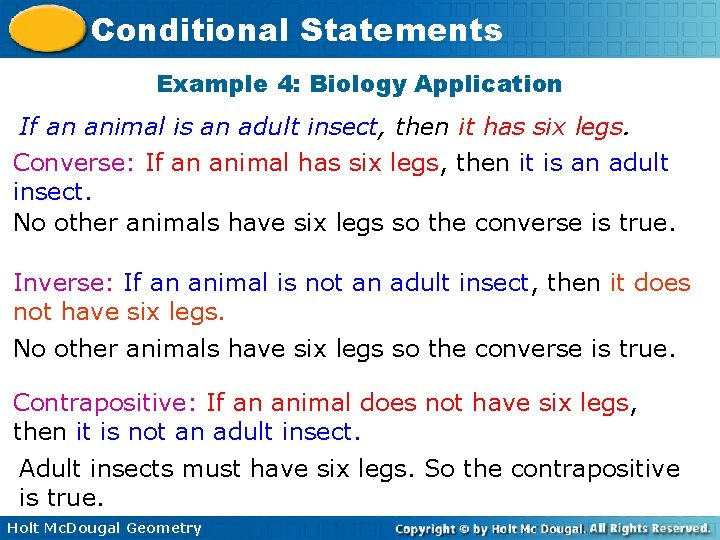Conditional Statements Example 4: Biology Application If an animal is an adult insect, then it has six legs. Converse: If an animal has six legs, then it is an adult insect. No other animals have six legs so the converse is true. Inverse: If an animal is not an adult insect, then it does not have six legs. No other animals have six legs so the converse is true. Contrapositive: If an animal does not have six legs, then it is not an adult insect. Adult insects must have six legs. So the contrapositive is true. Holt Mc. Dougal GeometryConditional Statements Check It Out! Example 4 Write the converse, inverse, and contrapostive of the conditional statement “If an animal is a cat, then it has four paws. ” Find the truth value of each. If an animal is a cat, then it has four paws. Holt Mc. Dougal Geometry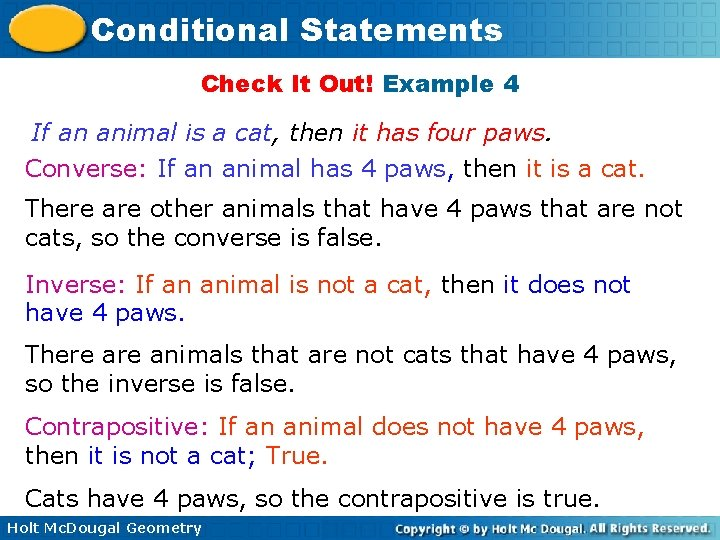Conditional Statements Check It Out! Example 4 If an animal is a cat, then it has four paws. Converse: If an animal has 4 paws, then it is a cat. There are other animals that have 4 paws that are not cats, so the converse is false. Inverse: If an animal is not a cat, then it does not have 4 paws. There animals that are not cats that have 4 paws, so the inverse is false. Contrapositive: If an animal does not have 4 paws, then it is not a cat; True. Cats have 4 paws, so the contrapositive is true. Holt Mc. Dougal GeometryConditional Statements Related conditional statements that have the same truth value are called logically equivalent statements. A conditional and its contrapositive are logically equivalent, and so are the converse and inverse. Holt Mc. Dougal Geometry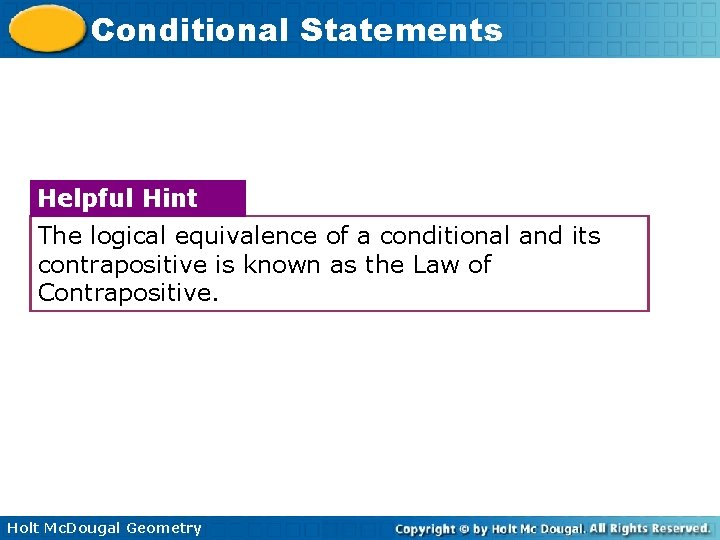Conditional Statements Helpful Hint The logical equivalence of a conditional and its contrapositive is known as the Law of Contrapositive. Holt Mc. Dougal Geometry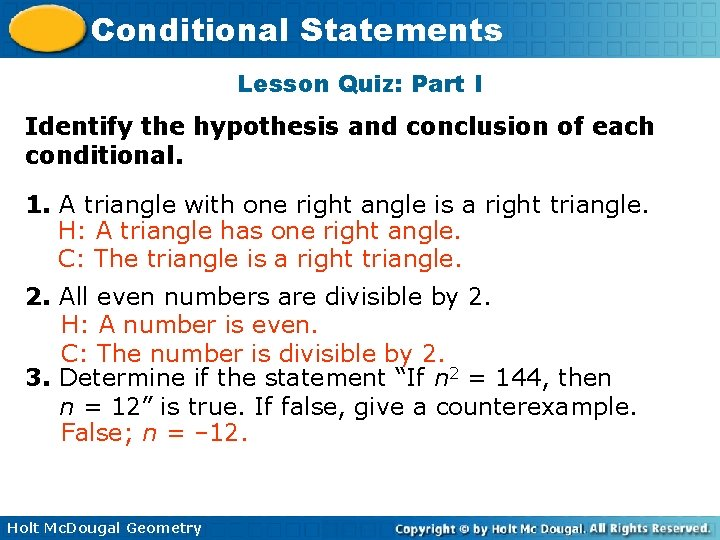Conditional Statements Lesson Quiz: Part I Identify the hypothesis and conclusion of each conditional. 1. A triangle with one right angle is a right triangle. H: A triangle has one right angle. C: The triangle is a right triangle. 2. All even numbers are divisible by 2. H: A number is even. C: The number is divisible by 2. 3. Determine if the statement “If n 2 = 144, then n = 12” is true. If false, give a counterexample. False; n = – 12. Holt Mc. Dougal Geometry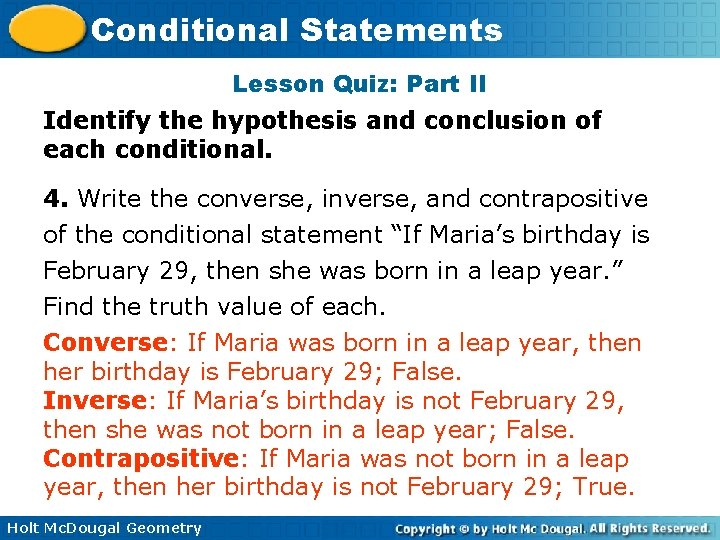Conditional Statements Lesson Quiz: Part II Identify the hypothesis and conclusion of each conditional. 4. Write the converse, inverse, and contrapositive of the conditional statement “If Maria’s birthday is February 29, then she was born in a leap year. ” Find the truth value of each. Converse: If Maria was born in a leap year, then her birthday is February 29; False. Inverse: If Maria’s birthday is not February 29, then she was not born in a leap year; False. Contrapositive: If Maria was not born in a leap year, then her birthday is not February 29; True. Holt Mc. Dougal Geometry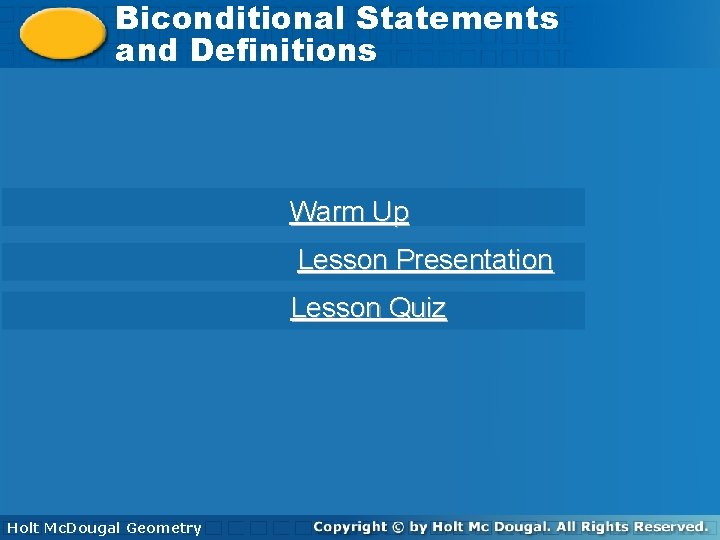Biconditional Statements Conditional Statements and Definitions Warm Up Lesson Presentation Lesson Quiz Holt Mc. Dougal Geometry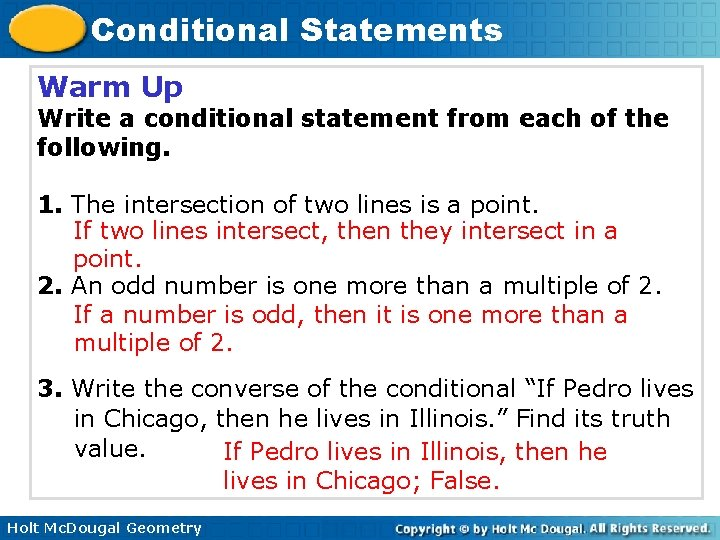Conditional Statements Warm Up Write a conditional statement from each of the following. 1. The intersection of two lines is a point. If two lines intersect, then they intersect in a point. 2. An odd number is one more than a multiple of 2. If a number is odd, then it is one more than a multiple of 2. 3. Write the converse of the conditional “If Pedro lives in Chicago, then he lives in Illinois. ” Find its truth value. If Pedro lives in Illinois, then he lives in Chicago; False. Holt Mc. Dougal Geometry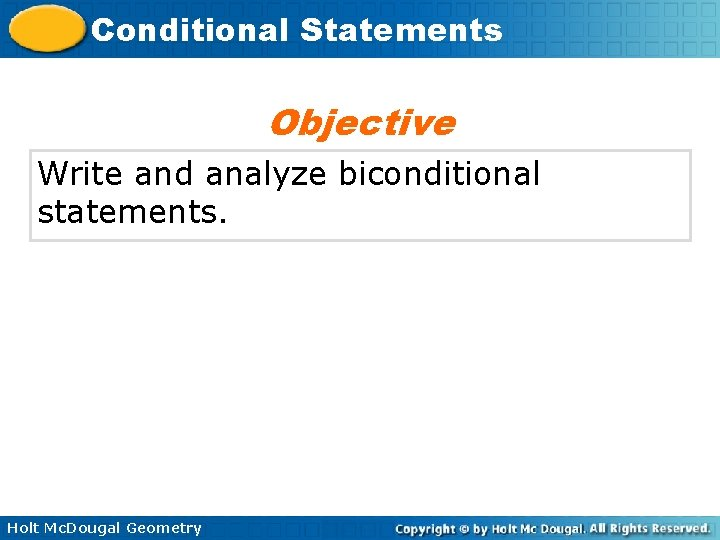Conditional Statements Objective Write and analyze biconditional statements. Holt Mc. Dougal Geometry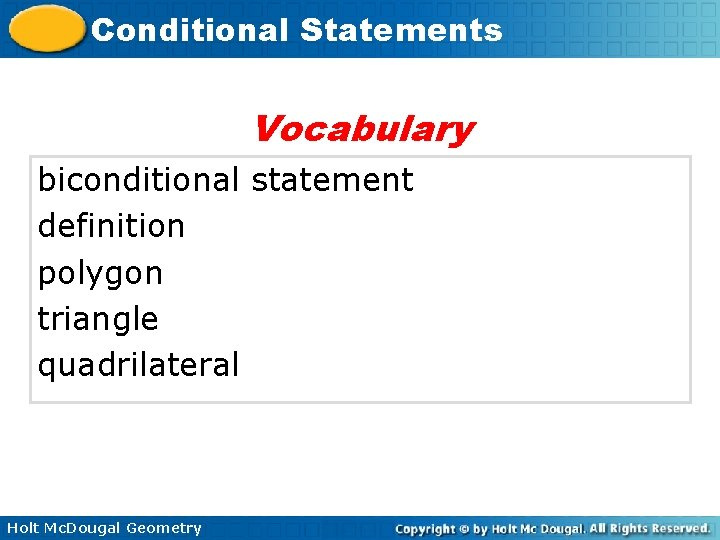Conditional Statements Vocabulary biconditional statement definition polygon triangle quadrilateral Holt Mc. Dougal GeometryConditional Statements When you combine a conditional statement and its converse, you create a biconditional statement. A biconditional statement is a statement that can be written in the form “p if and only if q. ” This means “if p, then q” and “if q, then p. ” Holt Mc. Dougal Geometry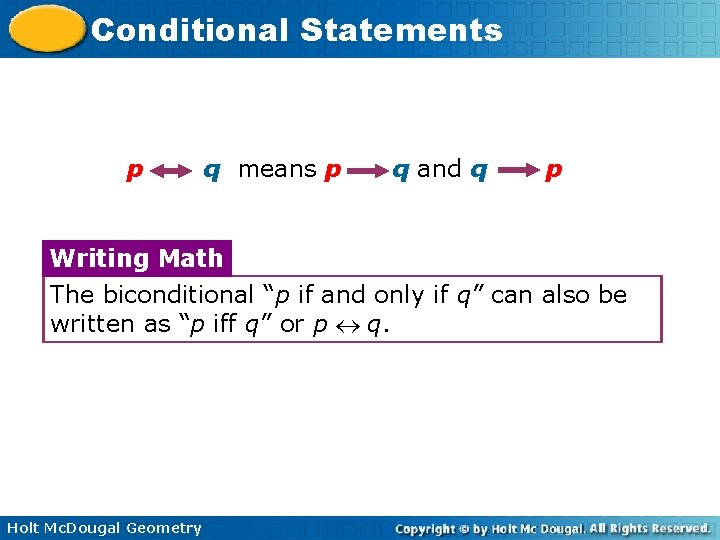Conditional Statements p q means p q and q p Writing Math The biconditional “p if and only if q” can also be written as “p iff q” or p q. Holt Mc. Dougal Geometry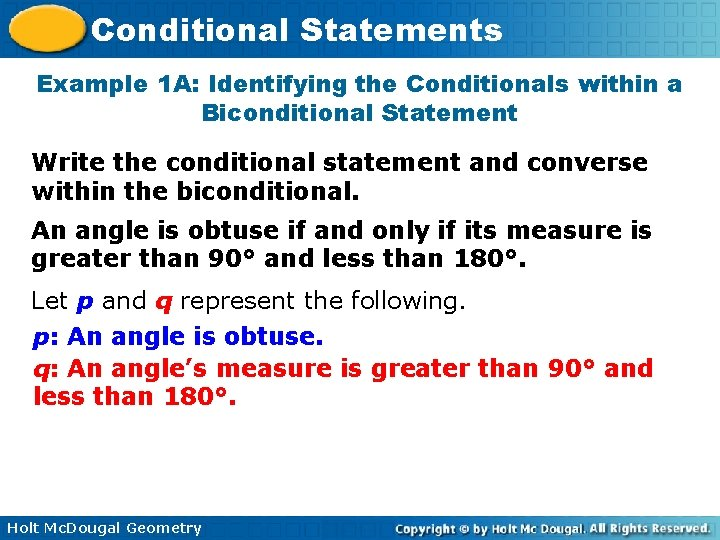Conditional Statements Example 1 A: Identifying the Conditionals within a Biconditional Statement Write the conditional statement and converse within the biconditional. An angle is obtuse if and only if its measure is greater than 90° and less than 180°. Let p and q represent the following. p: An angle is obtuse. q: An angle’s measure is greater than 90° and less than 180°. Holt Mc. Dougal GeometryConditional Statements Example 1 A Continued Let p and q represent the following. p: An angle is obtuse. q: An angle’s measure is greater than 90° and less than 180°. The two parts of the biconditional p q are p q and q p. Conditional: If an is obtuse, then its measure is greater than 90° and less than 180°. Converse: If an angle's measure is greater than 90° and less than 180°, then it is obtuse. Holt Mc. Dougal GeometryConditional Statements Example 1 B: Identifying the Conditionals within a Biconditional Statement Write the conditional statement and converse within the biconditional. A solution is neutral its p. H is 7. Let x and y represent the following. x: A solution is neutral. y: A solution’s p. H is 7. Holt Mc. Dougal Geometry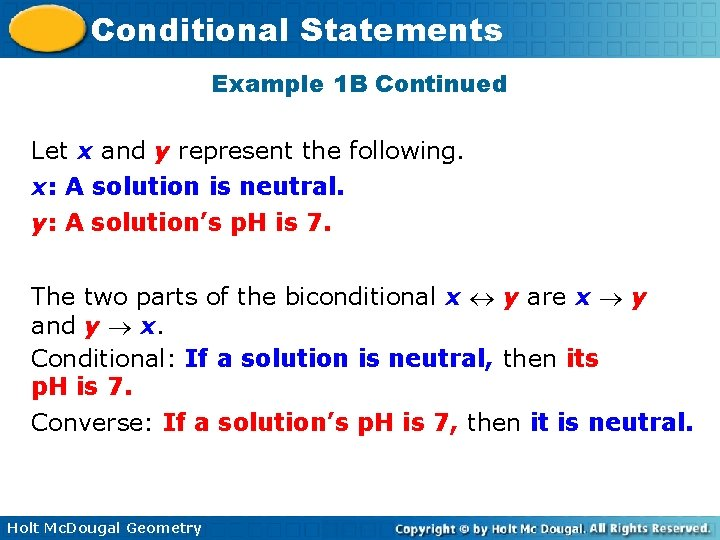Conditional Statements Example 1 B Continued Let x and y represent the following. x: A solution is neutral. y: A solution’s p. H is 7. The two parts of the biconditional x y are x y and y x. Conditional: If a solution is neutral, then its p. H is 7. Converse: If a solution’s p. H is 7, then it is neutral. Holt Mc. Dougal Geometry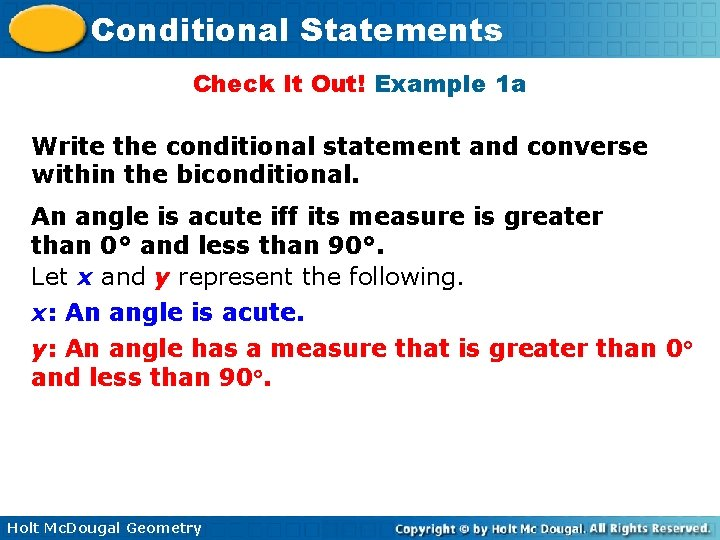Conditional Statements Check It Out! Example 1 a Write the conditional statement and converse within the biconditional. An angle is acute iff its measure is greater than 0° and less than 90°. Let x and y represent the following. x: An angle is acute. y: An angle has a measure that is greater than 0 and less than 90. Holt Mc. Dougal Geometry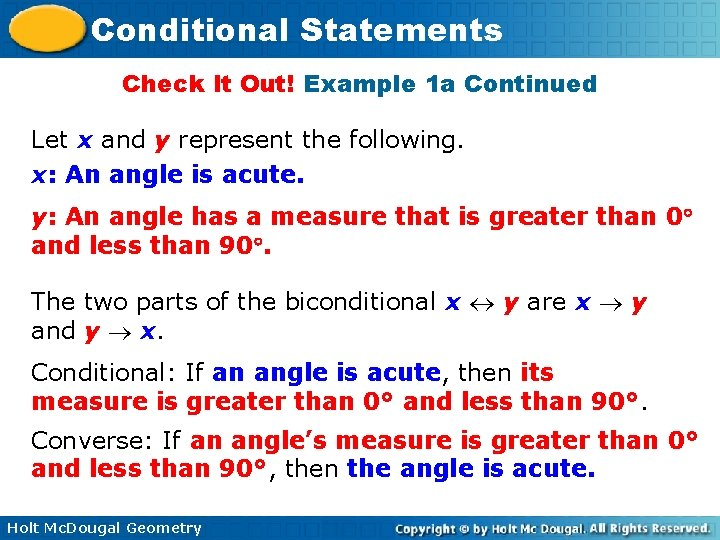Conditional Statements Check It Out! Example 1 a Continued Let x and y represent the following. x: An angle is acute. y: An angle has a measure that is greater than 0 and less than 90. The two parts of the biconditional x y are x y and y x. Conditional: If an angle is acute, then its measure is greater than 0° and less than 90°. Converse: If an angle’s measure is greater than 0° and less than 90°, then the angle is acute. Holt Mc. Dougal Geometry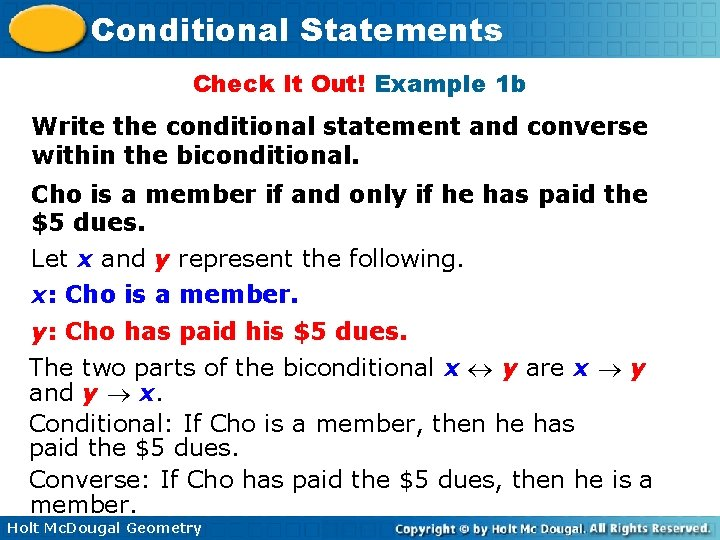Conditional Statements Check It Out! Example 1 b Write the conditional statement and converse within the biconditional. Cho is a member if and only if he has paid the \$5 dues. Let x and y represent the following. x: Cho is a member. y: Cho has paid his \$5 dues. The two parts of the biconditional x y are x y and y x. Conditional: If Cho is a member, then he has paid the \$5 dues. Converse: If Cho has paid the \$5 dues, then he is a member. Holt Mc. Dougal GeometryConditional Statements Example 2: Identifying the Conditionals within a Biconditional Statement For each conditional, write the converse and a biconditional statement. A. If 5 x – 8 = 37, then x = 9. Converse: If x = 9, then 5 x – 8 = 37. Biconditional: 5 x – 8 = 37 if and only if x = 9. B. If two angles have the same measure, then they are congruent. Converse: If two angles are congruent, then they have the same measure. Biconditional: Two angles have the same measure if and only if they are congruent. Holt Mc. Dougal Geometry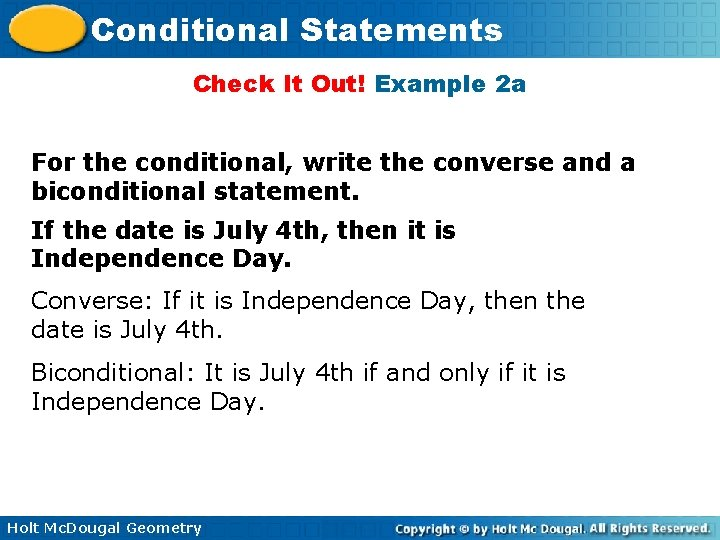Conditional Statements Check It Out! Example 2 a For the conditional, write the converse and a biconditional statement. If the date is July 4 th, then it is Independence Day. Converse: If it is Independence Day, then the date is July 4 th. Biconditional: It is July 4 th if and only if it is Independence Day. Holt Mc. Dougal Geometry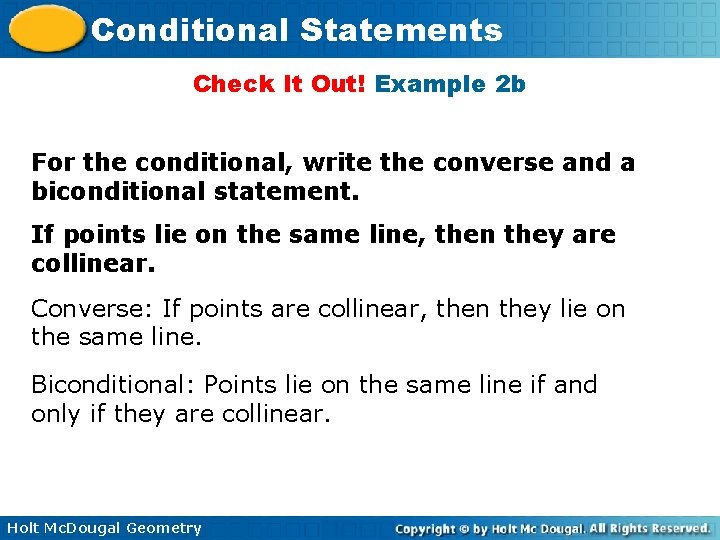Conditional Statements Check It Out! Example 2 b For the conditional, write the converse and a biconditional statement. If points lie on the same line, then they are collinear. Converse: If points are collinear, then they lie on the same line. Biconditional: Points lie on the same line if and only if they are collinear. Holt Mc. Dougal GeometryConditional Statements For a biconditional statement to be true, both the conditional statement and its converse must be true. If either the conditional or the converse is false, then the biconditional statement is false. Holt Mc. Dougal GeometryConditional Statements Example 3 A: Analyzing the Truth Value of a Biconditional Statement Determine if the biconditional is true. If false, give a counterexample. A rectangle has side lengths of 12 cm and 25 cm if and only if its area is 300 cm 2. Holt Mc. Dougal Geometry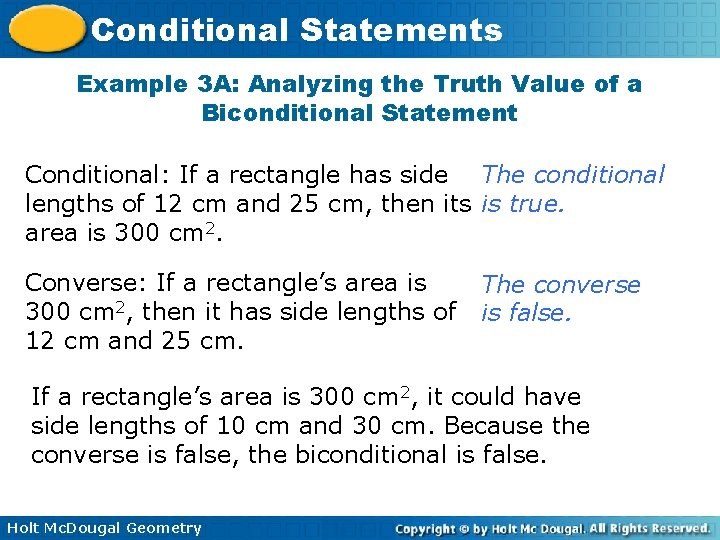Conditional Statements Example 3 A: Analyzing the Truth Value of a Biconditional Statement Conditional: If a rectangle has side The conditional lengths of 12 cm and 25 cm, then its is true. area is 300 cm 2. Converse: If a rectangle’s area is 300 cm 2, then it has side lengths of 12 cm and 25 cm. The converse is false. If a rectangle’s area is 300 cm 2, it could have side lengths of 10 cm and 30 cm. Because the converse is false, the biconditional is false. Holt Mc. Dougal Geometry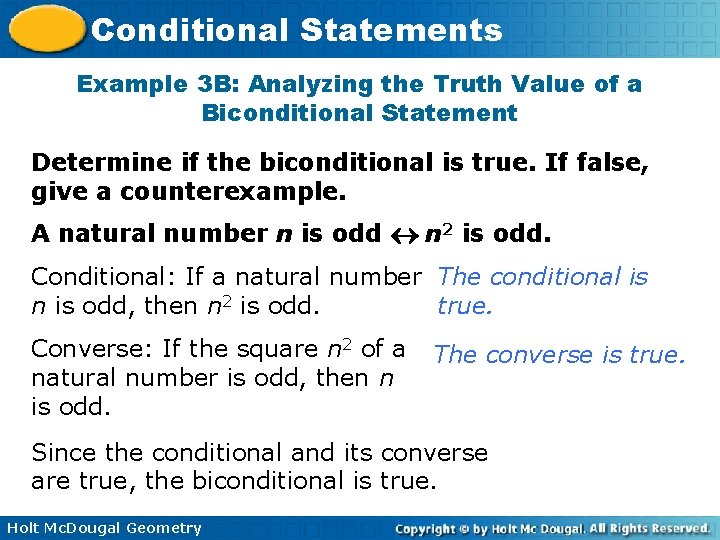Conditional Statements Example 3 B: Analyzing the Truth Value of a Biconditional Statement Determine if the biconditional is true. If false, give a counterexample. A natural number n is odd n 2 is odd. Conditional: If a natural number The conditional is n is odd, then n 2 is odd. true. Converse: If the square n 2 of a natural number is odd, then n is odd. The converse is true. Since the conditional and its converse are true, the biconditional is true. Holt Mc. Dougal Geometry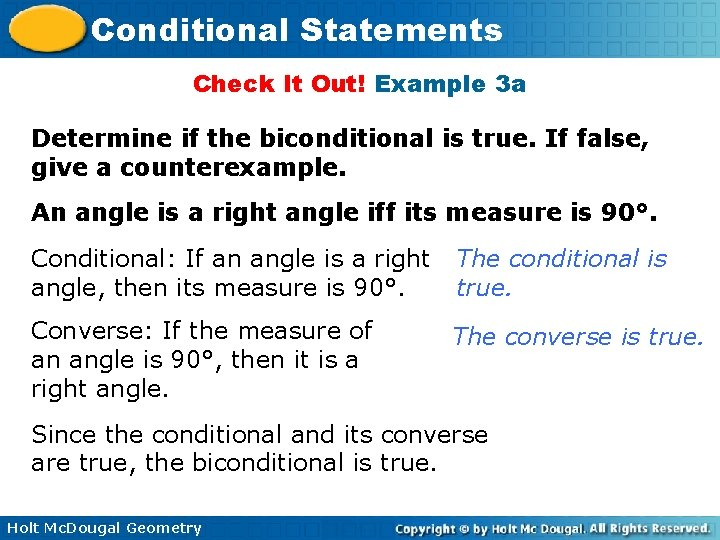Conditional Statements Check It Out! Example 3 a Determine if the biconditional is true. If false, give a counterexample. An angle is a right angle iff its measure is 90°. Conditional: If an angle is a right angle, then its measure is 90°. The conditional is true. Converse: If the measure of an angle is 90°, then it is a right angle. The converse is true. Since the conditional and its converse are true, the biconditional is true. Holt Mc. Dougal Geometry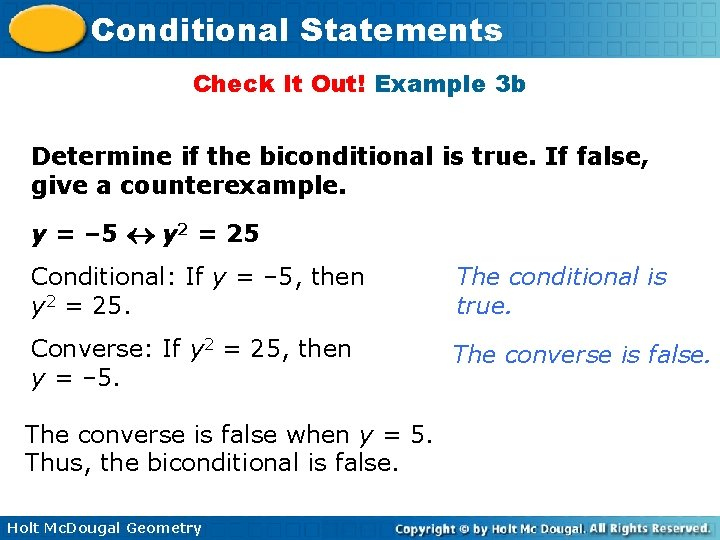Conditional Statements Check It Out! Example 3 b Determine if the biconditional is true. If false, give a counterexample. y = – 5 y 2 = 25 Conditional: If y = – 5, then y 2 = 25. The conditional is true. Converse: If y 2 = 25, then y = – 5. The converse is false when y = 5. Thus, the biconditional is false. Holt Mc. Dougal GeometryConditional Statements In geometry, biconditional statements are used to write definitions. A definition is a statement that describes a mathematical object and can be written as a true biconditional. Holt Mc. Dougal Geometry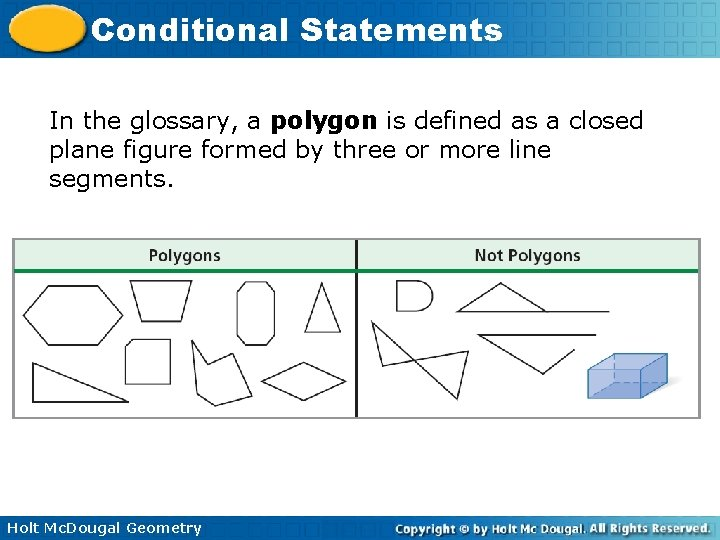Conditional Statements In the glossary, a polygon is defined as a closed plane figure formed by three or more line segments. Holt Mc. Dougal GeometryConditional Statements A triangle is defined as a three-sided polygon, and a quadrilateral is a four-sided polygon. Holt Mc. Dougal Geometry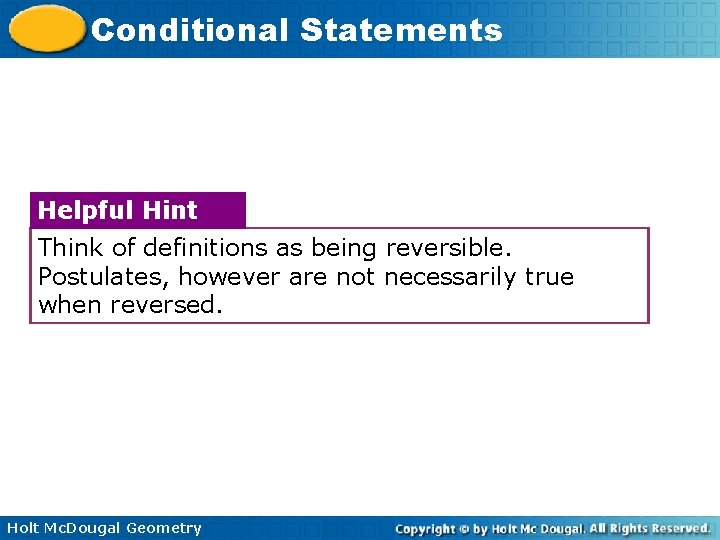Conditional Statements Helpful Hint Think of definitions as being reversible. Postulates, however are not necessarily true when reversed. Holt Mc. Dougal GeometryConditional Statements Example 4: Writing Definitions as Biconditional Statements Write each definition as a biconditional. A. A pentagon is a five-sided polygon. A figure is a pentagon if and only if it is a 5 -sided polygon. B. A right angle measures 90°. An angle is a right angle if and only if it measures 90°. Holt Mc. Dougal GeometryConditional Statements Check It Out! Example 4 Write each definition as a biconditional. 4 a. A quadrilateral is a four-sided polygon. A figure is a quadrilateral if and only if it is a 4 -sided polygon. 4 b. The measure of a straight angle is 180°. An is a straight if and only if its measure is 180°. Holt Mc. Dougal Geometry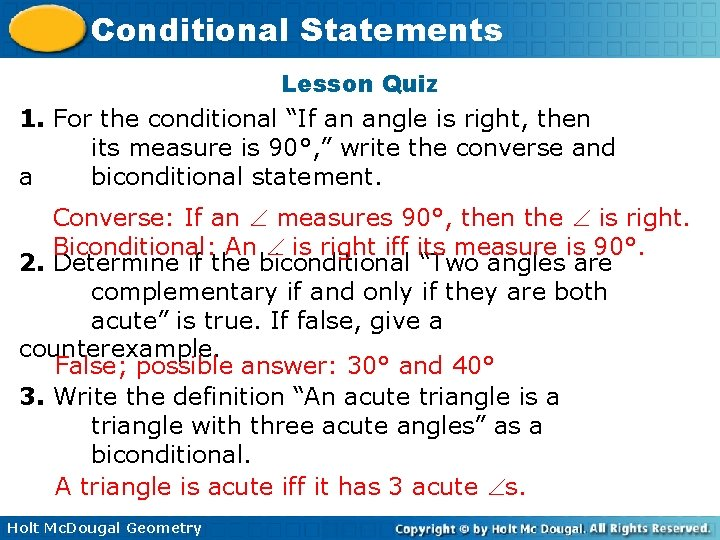Conditional Statements Lesson Quiz 1. For the conditional “If an angle is right, then its measure is 90°, ” write the converse and a biconditional statement. Converse: If an measures 90°, then the is right. Biconditional: An is right iff its measure is 90°. 2. Determine if the biconditional “Two angles are complementary if and only if they are both acute” is true. If false, give a counterexample. False; possible answer: 30° and 40° 3. Write the definition “An acute triangle is a triangle with three acute angles” as a biconditional. A triangle is acute iff it has 3 acute s. Holt Mc. Dougal Geometry